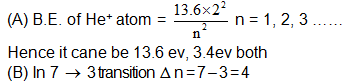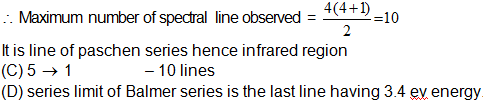Courses

# Atomic Structure MCQ - 2 (Advance)

## 20 Questions MCQ Test Chemistry for JEE Advanced | Atomic Structure MCQ - 2 (Advance)

Description
This mock test of Atomic Structure MCQ - 2 (Advance) for JEE helps you for every JEE entrance exam. This contains 20 Multiple Choice Questions for JEE Atomic Structure MCQ - 2 (Advance) (mcq) to study with solutions a complete question bank. The solved questions answers in this Atomic Structure MCQ - 2 (Advance) quiz give you a good mix of easy questions and tough questions. JEE students definitely take this Atomic Structure MCQ - 2 (Advance) exercise for a better result in the exam. You can find other Atomic Structure MCQ - 2 (Advance) extra questions, long questions & short questions for JEE on EduRev as well by searching above.
*Multiple options can be correct
QUESTION: 1

### If the wave number of 1st line of Balmer series of H-atom is 'x' then:

Solution: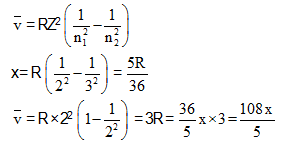*Multiple options can be correct
QUESTION: 2

### wave functions of 3s and 3pz orbitals are given by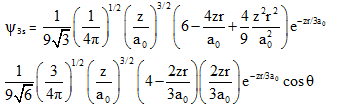from these we can conclude

Solution: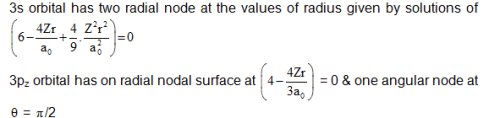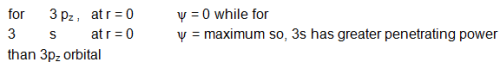*Multiple options can be correct
QUESTION: 3

### In a hydrogen like sample electron is in 2nd excited state, the Binding energy of 4th state of this sample is 13.6 eV, then

Solution: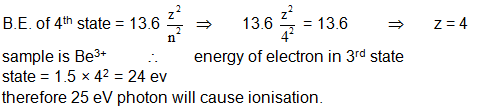*Multiple options can be correct
QUESTION: 4

1st  excitation potential for the H-like (hypothetical) sample is 24 V. Then:

Solution: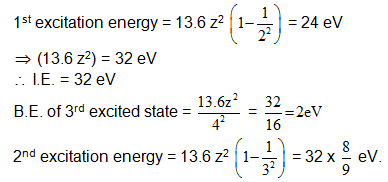*Multiple options can be correct
QUESTION: 5

A hydrogen like atom in ground state absorbs 'n' photons having the same energy and it emits exactly 'n' photons when electronic transition takes place. Then the energy of the absorbed photon may be

Solution: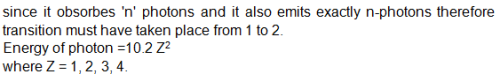*Multiple options can be correct
QUESTION: 6

In a hydrogen like sample two different types of photons A and B are produced by electronic transition. Photon B has it's wavelength in infrared region if photon A has more energy then B, then the photon A may belong to the region.

Solution:

Since B is in infrared region and A has more energy than B hence it will have lesser wave length i.e. ultra violet, visible or infrared region

*Multiple options can be correct
QUESTION: 7

Hydrogen atoms in a particular excited state 'n', when all returned to ground state, 6 different photons are emitted. This of the following is/are incorrect.

Solution:
*Multiple options can be correct
QUESTION: 8

In a H-like sample electrons make transition from 4th excited state to 2nd state then

Solution:
QUESTION: 9

Which of the following ion has highest magnetic moment.

Solution: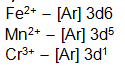Clearly Mn2 has maximum number of unpaired electrons therefore it has highest magnetic moment.

QUESTION: 10

If an ion of 25Mn has a magnetic moment of 3.873 B. M. Then Mn is in which state.

Solution: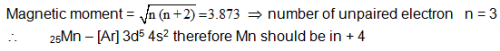QUESTION: 11

Which of the following is a paramagnetic substance.

Solution: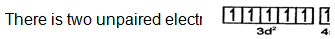QUESTION: 12

The number of unpaired electrons in Mn4+ (Z = 25) is –

Solution: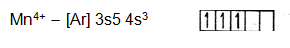QUESTION: 13

If proton, electron and a-particle are moving with same kinetic energy then the order of their de-Broglie's wavelength.

Solution:
QUESTION: 14

Using Bohr's theory, the transition, so that the electrons de-Broglie wavelength becomes 3 times of its original value in He+ ion will be

Solution:
QUESTION: 15

De-Broglie wavelength of an electron travelling with speed equal to 1% of the speed of light

Solution:
QUESTION: 16

If an electron is in any higher state n = n and makes a transition to ground state, then total no. of different photons emitted is equal to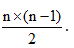If an electrons is in any higher state n = n2 and makes a transition to another excited state n = n1, then total no. of different photons emitted is equal to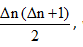where Δ n = n 2 – n 1 .

Note: In case of single isolated atom if electron make transition from nth state to the ground state then max. number of spectral lines observed = ( n – 1).

Q.

Two samples are taken, in 1st sample mole ratio of H to H+ is 1: 2. In IInd sample mole ratio of H to He+ is 2: 1. If n1 is number of spectral lines produced by 1st sample and number of spectral lines produced by lino sample is n2 then n1: n2 will be – (There is sufficient number of both types of atom and both are in same excited state in both sample)

Solution:
QUESTION: 17

Which of the following statements is/are true?

(I) If H atoms are in 3rd state then number of maximum spectral line produced by 2 atoms will be equal to number of maximum spectral lines produced by 3 H-atoms

(II) For third excited state, maximum number of spectral line produced by He+ and maximum number of spectral line produced by 4 H - atom are not equal.

(III) Energy evolved from 3 to 2 transitions in He cannot used for any transition in Li2+.

Solution:
QUESTION: 18

If an electron is in any higher state n = n and makes a transition to ground state, then total no. of different photons emitted is equal toIf an electrons is in any higher state n = n2 and makes a transition to another excited state n = n1, then total no. of different photons emitted is equal towhere Δ n = n 2 – n 1 .

Note: In case of single isolated atom if electron make transition from nth state to the ground state then max. number of spectral lines observed = ( n – 1).

Q.

In a sample one H atom is in 1st excited state, two He+ ions is in IInd excited state and three Li2+ ions is in Illrd excited state are present then maximum, number of spectral line which can be obtained when all possible transition terminated at n = 1.

Solution:
QUESTION: 19

Pn = potential energy, En = total energy

f = frequency, Z = atomic number

vn = velocity in nth orbit

Tn = time period in nth orbit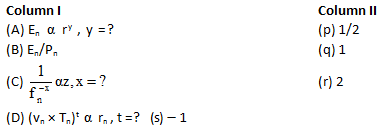Solution: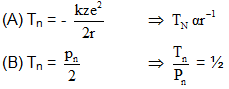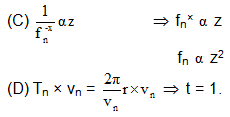QUESTION: 20

Match the following

B.E. - Binding energy

I.E. - Ionization energy: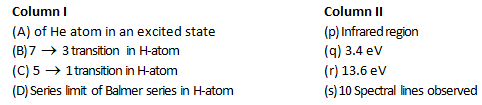Solution: